# Week 3

(notes from the lighthouse3d tutorial and the Orange book)

### But first lets back up a bit and talk about functions in shaders some more

GLSL uses call by value-return
There are no pointers
- input parameters will be copied into the function (no passing by reference)
- output parameters will be copied back out of the function to the caller

- in (implied) - copy in but dont copy back out, writable within the function
- const (const in) - same as in, but not writable within the function
- out - copy out, undefined at entry to the function
- inout - copy in and copy out

### Lighting

Overview (from the nice lighthouse3d people)
1. The OpenGL application sends a color using the glColor function
2. The vertex shader receives the color value in the attribute gl_Color
3. The vertex shader computes the front face and back face colors, and stores them in gl_FrontColor, and gl_BackColor respectively
4. The fragment shader receives an interpolated color in the varying variable gl_Color, depending on the orientation of the current primitive, i.e. the interpolation is done using either the gl_FrontColor or the gl_BackColor values.
5. The fragment shader sets gl_FragColor based on the value of gl_Color

From week 2 of class we have the following built-in varying variables:

varying vec4 gl_FrontColor;
varying vec4 gl_BackColor;
varying vec4 gl_FrontSecondaryColor;
varying vec4 gl_BackSecondaryColor;

varying vec4 gl_Color;
varying vec4 gl_SecondaryColor;

So what's with these SecondaryColors?
We can compute the colour at a vertex in 1 or 2 parts if we want. By default the primary colour is computed from emissive, ambient, diffuse, and specular. If there is a secondary colour then the primary colour is computed from emissive, ambient, and diffuse while the secondary colour has the specular component (to allow the specular highlights to be applied seperately after texturing so they match the colour of the light source.) For now we'll do without it to keep things simple.

No Lights

pass the per-vertex colour and the secondary colour along

if (secondaryColor)
gl_FrontSecondaryColor = gl_SecondaryColor;

gl_FrontColor = gl_Color;

There is a bunch of code in the Orange Book (1st or 2nd Ed.) on how to do lighting in Chapter 9, which is a very good reference for doing things right, but I think its overly complex to start out with. So instead we are going back to the nice lighthouse3d people and looking at their lighting tutorial for directional lights with GLSL.

http://www.lighthouse3d.com/tutorials/glsl-tutorial/lighting/

Fog

Fog is used to simulate atmospheric effects that make objects appear less distinct the further they are away from the camera. Its often used in combination with the far clipping plane to hide far away objects popping in and out of the scene. OpenGL fog has an RGB colour which typically matches the background colour. The fog colour is used to modify the final colour of objects with several different possible equations for how the object colour and for colour are mixed (linear, exponential, more exponential.) With linear fog the fog starts (fog=0%) at a given distance from the camera, ends (fog=100%) at a further distance from the camera, with linear mapping in between. With exponential fog a fog density is used along with the distance

From week 2 of class we have the following built-in varying variable:

varying float gl_FogFragCoord;

attribute float gl_FogCoord; // vertex attribute like gl_Normal or gl_Vertex

in the simple case the vertex shader will do
gl_FogFragCoord = gl_FogCoord;

Typically compute in the vertex shader and then pass to the fragment shader since its faster but you could compute fog in the fragment shader

fog = (gl_Fog.end - gl_FogFragCoord) * gl_Fog.scale; // linear

fog = exp2(-gl_Fog.density * gl_FogFragCoord * 1.442695); // exponential

fog = exp2(-gl_Fog.density * gl_FogDensity
* gl_FogFragCoord * gl_FogFragCoord * 1.442695); // more exponential

where gl_Fog.scale is 1.0 / (gl_Fog.end - gl_Fog.start)

then clamp with

fog = clamp( fog, 0.0, 1.0);

color = mix(vec3 (gl_Fog.color), color, fog);

Textures

Texture Access Functions:
vec4 texture2D(sampler2D sampler, vec2 coord)
use coord to do a lookup into the texture currently specified by the sampler

A typical 2D texture is created or leaded into the program with a certain number of pixels in either dimension. For better speed its highly suggested that the texture be square and have the number of pixels in each dimension be a power of 2 (e.g. 16, 32, 64, 128, 256, 512, 1024, etc) so a nice texture size is 512 x 512 or 1024 x 1024. Internally the texture is indexed by (s, t) values which range from 0.0 to 1.0 allowing textures with different numbers of pixels to use the same coordinate system.

When we specify the vertex of a polygon we can give that vertex a location, a normal vector, a color, and a texture coordinate. In the example below we are in immediate mode specifying the values in turn. As we saw back in week 1 the more modern way makes use of arrays of vertices, colours, textures.

in the application program:

for example we could initialize a given texture with the following function:

GLuint textureNum;         // space to hold one texture name
glGenTextures(1, textureNum); // generate one unique texture name

// create new 2D texture and give it the unique name generated above
// more info on all this in chapter 9 (Texture Mapping) of the red book
glBindTexture(GL_TEXTURE_2D, textureNum);

// define a new texture - lots of possible parameter values here
// here are a couple typical ones: greyscale and rgb
//
// 2D texture
// not doing multi-resolution so level=0
// internal format = 1 (GL_LUMINANCE) only one value per texel
// width and height both = 64
// no border so border = 0
// format = GL_LUMINANCE
// type = unsigned byte
// pointer to where the texture data was stored after being read in
glTexImage2D(GL_TEXTURE_2D, 0, 1, 64, 64, 0, GL_LUMINANCE,
GL_UNSIGNED_BYTE, (const GLvoid *) texData);

// or a 512 by 512 rgb texture
//glTexImage2D(GL_TEXTURE_2D, 0, GL_RGB, 512, 512, 0, GL_RGB,
//    GL_UNSIGNED_BYTE, (const GLvoid *) texData);

// if we give s,t values outside the 0-1 bounds we want to wrap around
glTexParameterf(GL_TEXTURE_2D, GL_TEXTURE_WRAP_S, GL_REPEAT);
glTexParameterf(GL_TEXTURE_2D, GL_TEXTURE_WRAP_T, GL_REPEAT);

// how do we deal with interpolation
glTexParameterf(GL_TEXTURE_2D, GL_TEXTURE_MAG_FILTER, GL_LINEAR);
glTexParameterf(GL_TEXTURE_2D, GL_TEXTURE_MIN_FILTER, GL_LINEAR);

// and then save off the texture name so we can use it later
texStore = textureNum;

and then we could draw the polygon to be texture mapped as normal:

glActiveTexture(GL_TEXTURE0);
glBindTexture(GL_TEXTURE_2D, texStore);

glBegin( GL_POLYGON );
// set the s and t texture coordinates for this vertex
glTexCoord2f( 1.0f, 1.0f );
// we could also set a colour and/or a normal for this vertex
// and here is the vertex
glVertex3f( -2.0f, -2.0f, 0.0f);

// now we set up the information for the next vertex
glTexCoord2f( 0.0f, 1.0f );
glVertex3f(  2.0f, -2.0f, 0.0f);

// and the third vertex
glTexCoord2f( 0.0f, 0.0f );
glVertex3f(  2.0f,  2.0f, 0.0f);

// and the fourth vertex
glTexCoord2f( 1.0f, 0.0f );
glVertex3f( -2.0f,  2.0f, 0.0f);
glEnd();

we need two uniform variables - light position for vertex shader and texture unit for fragment shader. This should look similar to the procedural brick example that we went through last week.

GLint texLoc, lightLoc;
texLoc = glGetUniformLocation(programObj, "textureName");
glUniform1i(texLoc, 0); // 0 because first texture is number 0, second is 1, third is 2, etc

lightLoc = glGetUniformLocation(programObj, "LightPosition");
glUniform3f(lightLoc, 0.0, 0.0, 4.0);

varying float LightIntensity;
uniform vec3 LightPosition;

const float specularContribution = 0.1;
const float diffuseContribution = 1.0 - specularContribution;

varying vec2 TexCoord;

void main(void)
{
vec3 ecPosition = vec3 (gl_ModelViewMatrix * gl_Vertex);
vec3 tnorm      = normalize(gl_NormalMatrix * gl_Normal);
vec3 lightVec   = normalize(LightPosition - ecPosition);
vec3 reflectVec = reflect(-lightVec, tnorm);
vec3 viewVec    = normalize(-ecPosition);

float spec = clamp(dot(reflectVec, viewVec), 0.0, 1.0);
spec = pow(spec, 16.0);

LightIntensity = diffuseContribution * max(dot(lightVec, tnorm), 0.0)
+ specularContribution * spec;

// get the texture coordinates from the OpenGL program and
// store it in the varying variable TexCoord for the fragment shader
// current versions of glsl have the built in varying variable gl_TexCoord[i]
// so you could also write gl_TexCoord  = gl_MultiTexCoord0;
TexCoord = gl_MultiTexCoord0.st;
gl_Position = ftransform();
}

varying float LightIntensity;
uniform sampler2D textureName;
varying vec2 TexCoord;

void main(void)
{
// use the interpolated s and t values to grab the colour
// from the appropriate location in the texture
// the more modern usage with
gl_TexCoord would be
//
vec3 texColor = vec3 (texture2D (textureName, TexCoord.st));
vec3 texColor = vec3 (texture2D (textureName, TexCoord.st));
gl_FragColor  = vec4 (texColor * LightIntensity, 1.0);
}

note that this is a very simple case where we are using the colour of the texture and the light intensity to give the final colour. We are not trying to blend the texture with the colour of the underlying fragment.

in an even simpler case we could just assign the colour of the fragment to be the colour of the texture at that location and ignore the light:

gl_FragColor = vec4 (texColor, 1.0);

we can also try to blend the texture colour with the underlying primitive colour which is affected by the light:
void main(void)
{
vec4 texel     = texture2D (textureName, TexCoord.st);
vec3 texColor  = texel.rgb;
float texAlpha = texel.a;

vec3 objColor  =
LightIntensity * gl_FrontMaterial.diffuse.rgb +
gl_FrontMaterial.ambient.rgb;
float objAlpha = gl_FrontMaterial.diffuse.a;

gl_FragColor   = vec4 (texColor * objColor, texAlpha * objAlpha);
}

we could also play with the channels of the texture - i.e. showing only the red component:
gl_FragColor    = vec4 (texColor.r, 0.0, 0.0, 1.0);

Here you are doing what OpenGL will do automatically for you with GL_REPLACE and GL_MODULATE. As above with the lighting it gives you a better idea what is going on behind the scenes and why some settings in OpenGL take longer to render than others.

Multitexturing

Its pretty simple using GLSL to combine two textures together. The fragment shader needs a new sampler for the second texture:

uniform sampler2D textureName0, textureName1;

if we use the same texture coordinates for both textures then we can do the following, which shows the red channel of the first texture in red combined with showing the red channel of the second texture in blue:

vec3 texColor0 = vec3 (texture2D (textureName0, TexCoord.st));
vec3 texColor1 = vec3 (texture2D (textureName1, TexCoord.st));

gl_FragColor   = vec4 (texColor0.r * LightIntensity, 0.0,
texColor1.r * LightIntensity, 1.0);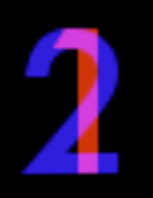otherwise we would need specific texture coordinates for the second texture. In this case we would need the vertex shader to set two varying variables - one to hold s and t values for the first texture, and another to hold s and t values for the second texture.
varying vec2 TexCoord0, TexCoord1;
TexCoord0 = gl_MultiTexCoord0.st;
TexCoord1 = gl_MultiTexCoord1.st;

and then the fragment shader can do:

vec4 texel = texture2D (textureName0, TexCoord0.st) +

texture2D (textureName1, TexCoord1.st);

The syntax for this is changing again in version 140, so if you look in the 3rd edition of the Orange Book in chapter 10 you will see some slightly different code.

You could also dynamically change the blending of the textures based on say time, or lighting. This allows you to do simple animations.

The Day/Night Earth example from Chapter 10 of either the 1st or 2nd editions of the Orange Book is another good example.

Environment Mapping

Modelling reflections in a complex environment without 'doing it right' using ray-tracing

Take a single texture map covering 360 degrees horizontal and 180 degrees vertical or 6 textures forming a cube map.

For a single texture map we use the reflection vector from an object's surface
(converted to altitude and azimuth) to get colour from the spherical environment map. In this case we are generating the texture coordinates from within the fragment shader for each fragment.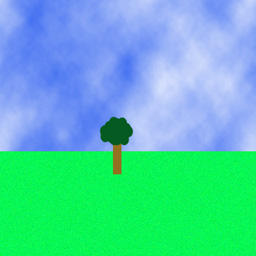in the Application Program:

set up the uniform variables:
LightPos - vec3 - location of the light source in eye coordinates
BaseColor - vec3 - base colour of the object
MixRatio - float - ratio of base colour to environment map reflection
envMap - sampler2D - which texture unit the environment map is stored in

// Vertex shader for environment mapping with an
// equirectangular 2D texture
// Authors: John Kessenich, Randi Rost
// Copyright (c) 2002-2004 3Dlabs Inc. Ltd.

varying vec3 Normal;          // surface normal in eye coordinates
varying vec3 EyeDir;          // eye direction == loc of vertex in eye coordinates
// (the eye is at 0,0,0 in canonical view volumes)
varying float LightIntensity; // diffuse light

uniform vec3 LightPos;

void main (void)
{
gl_Position    = ftransform();
Normal         = normalize(gl_NormalMatrix * gl_Normal);
vec4 pos       = gl_ModelViewMatrix * gl_Vertex;
EyeDir         = pos.xyz;
LightIntensity = max(dot(normalize(LightPos - EyeDir), Normal), 0.0); // diffuse lighting
}

// Fragment shader for environment mapping with an
// equirectangular 2D texture
// Authors: John Kessenich, Randi Rost
// Copyright (c) 2002-2004 3Dlabs Inc. Ltd.

const vec3 Xunitvec = vec3 (1.0, 0.0, 0.0);
const vec3 Yunitvec = vec3 (0.0, 1.0, 0.0);

uniform vec3 BaseColor;
uniform float MixRatio;

uniform sampler2D envMap;

varying vec3 Normal
varying vec3 EyeDir;
varying float LightIntensity;

void main (void)
{
// compute reflection vector
vec3 reflectDir = reflect(EyeDir, Normal);

// compute altitude and azimuth angles from reflection vector

vec2 index;

index.y = dot(normalize(reflectDir), Yunitvec);       // altitude
reflectDir.y = 0.0;
index.x = dot(normalize(reflectDir), Xunitvec) * 0.5; // azimuth

// index.x ranges from -0.5 to 0.5
// index.y ranges from -1 to 1
// texture coordinates range from 0 to 1 in both dimensions (aside from wrapping)

// Translate index values into proper range
// t (elevation) value is straight forward, s (azimuth) is a bit more complicated
// if reflectDir.z >= 0.0, s will range from 0.25 to 0.75 (front side)
// if reflectDir.z  < 0.0, s will range from 0.75 to 1.25 (-0.25) (back side)
//                                           which is OK since we are wrapping
// t will range from 0 to 1

if (reflectDir.z >= 0.0) // reflecting towards the front
index = (index + 1.0) * 0.5;

else                     // reflecting towards the back

{
index.s = (-index.s) * 0.5 + 1.0;
index.t = (index.t + 1.0) * 0.5; // same as for index.t above
}

// Do a lookup into the environment map
vec3 envColor = vec3 (texture2D(envMap, index));

// Add lighting to base colour and mix
vec3 base = LightIntensity * BaseColor;
envColor = mix(envColor, base, MixRatio);

gl_FragColor = vec4(envColor, 1.0);

}

and the result is the following:

Here is some sample code for the spherical environment map: application, vertex shader, fragment shader and here is a gzipped tar file with all the parts

One could also use this kind of simple environment map on a more complex shape like this .obj file of my head that now has mars reflected on it:For a cube map we have the following set of planes defining the environment map: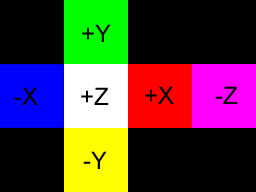So for example we could have the following simple set of 6 textures forming a cube map where each texture is a solid colour. In this case they are 64 by 64 pixels.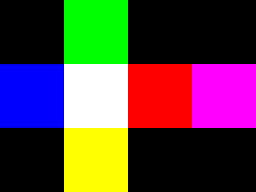you would then use vec4 textureCube (samplerCube sampler, vec3 coord) to do the lookup and the code simplifies quite a bit to the following:

vec3 BaseColor = vec3(1.0, 1.0, 1.0);
float MixRatio = 0.5;

varying vec3 Normal;
varying vec3 EyeDir;
varying float LightIntensity;

uniform samplerCube envMap; // which will be 0 in this case

void main (void)
{
// compute reflection vector
vec3 reflectDir = reflect(EyeDir, Normal);

// Do a lookup into the environment map
vec3 envColor = vec3 (textureCube( envMap, normalize(reflectDir)));

// Add lighting to base colour and mix
vec3 base = LightIntensity * BaseColor;
envColor = mix(envColor, base, MixRatio);

gl_FragColor = vec4(envColor, 1.0);
}

but you have the extra work of setting up the cube map in the main OpenGL application:

GLuint textureNum;
glGenTextures(1, textureNum);

glActiveTexture(GL_TEXTURE0);
glBindTexture(GL_TEXTURE_CUBE_MAP, textureNum);

glTexParameteri(GL_TEXTURE_CUBE_MAP, GL_TEXTURE_WRAP_S, GL_REPEAT);
glTexParameteri(GL_TEXTURE_CUBE_MAP, GL_TEXTURE_WRAP_T, GL_REPEAT);
glTexParameteri(GL_TEXTURE_CUBE_MAP, GL_TEXTURE_WRAP_R, GL_REPEAT);

glTexParameteri(GL_TEXTURE_CUBE_MAP, GL_TEXTURE_MAG_FILTER, GL_LINEAR);
glTexParameteri(GL_TEXTURE_CUBE_MAP, GL_TEXTURE_MIN_FILTER, GL_LINEAR);

glTexImage2D(GL_TEXTURE_CUBE_MAP_POSITIVE_X,
0, GL_RGB, 64, 64, 0, GL_RGB, GL_UNSIGNED_BYTE, &redData);
glTexImage2D(GL_TEXTURE_CUBE_MAP_NEGATIVE_X,
0, GL_RGB, 64, 64, 0, GL_RGB, GL_UNSIGNED_BYTE, &blueData);

glTexImage2D(GL_TEXTURE_CUBE_MAP_POSITIVE_Y,
0, GL_RGB, 64, 64, 0, GL_RGB, GL_UNSIGNED_BYTE, &greenData);
glTexImage2D(GL_TEXTURE_CUBE_MAP_NEGATIVE_Y,
0, GL_RGB, 64, 64, 0, GL_RGB, GL_UNSIGNED_BYTE, &yellowData);

glTexImage2D(GL_TEXTURE_CUBE_MAP_POSITIVE_Z,
0, GL_RGB, 64, 64, 0, GL_RGB, GL_UNSIGNED_BYTE, &whiteData);
glTexImage2D(GL_TEXTURE_CUBE_MAP_NEGATIVE_Z,
0, GL_RGB, 64, 64, 0, GL_RGB, GL_UNSIGNED_BYTE, &purpleData);

The result of cube mapping the typical glut teapot with the above cubemap is shown below:

Here is some sample code for cube mapping: application, vertex shader, fragment shader
and here is a gzipped tar file of all the parts

you could also do refraction as well as reflection as discussed in chapter 14 of the 2nd edition. In this case we not only complute a reflection vector bouncing off the surface of the object but also a refraction vector that goes through the object. For more on refraction you can see: http://en.wikipedia.org/wiki/Index_of_refraction

// from the Orange Book 2nd edition

const float Eta = 0.66;
const float FresnelPower = 0.5;
const float F = ((1.0-Eta)*(1.0-Eta))/((1.0+Eta)*(1.0+Eta));

varying vec3 Reflect;
varying vec3 Refract;
varying float Ratio;

void main()
{
vec4 ecPosition = gl_ModelViewMatrix * gl_Vertex;
vec3 ecPosition3 = ecPosition.xyz / ecPosition.w;

vec3 i = normalize(ecPosition3);
vec3 n = normalize(gl_NormalMatrix * gl_Normal);

Ratio = F + (1.0 - F) * pow((1.0 - dot(-i, n)), FresnelPower);

Refract = refract(i, n, Eta);
Refract = vec3(gl_TextureMatrix * vec4 (Refract, 1.0));

Reflect = reflect(i, n);
Reflect = vec3(gl_TextureMatrix * vec4 (Reflect, 1.0));

gl_Position = ftransform();
}

// the code above does things a bit more properly taking the gl_TextureMatrix into account, though usually that will be the identity matrix.

// from the Orange Book 2nd edition

varying vec3 Reflect;
varying vec3 Refract;
varying float Ratio;

uniform samplerCube envMap;

void main (void)
{
vec3 refractColor = vec3(textureCube(envMap, Refract));
vec3 reflectColor = vec3(textureCube(envMap, Reflect));

vec3 color = mix(refractColor, reflectColor, Ratio);

gl_FragColor = vec4(color, 1.0);
}

here is an example with refraction turned on and the background, which was purple in the previous example, set to the squirrel photo we will use next week.and to be even fancier you can use different values of Eta for the R, G, and B components to get chromatic aberration - those colour fringes you see on cheap wide angle camera lenses - for example for a glass/air transition the values are R: 0.65, G: 0.67, and B: 0.69 with more info at  http://en.wikipedia.org/wiki/Chromatic_aberration

here is a gzipped tar file containing all the parts for that.

its hard to see chromatic aberration with the squirrel background, but with a white circle on a black background it becomes much more obvious. Below there is the reflecting teapot, a reflecting and refracting teapot without chromatic aberration, and finally a reflecting and refracting teapot with chromatic aberration.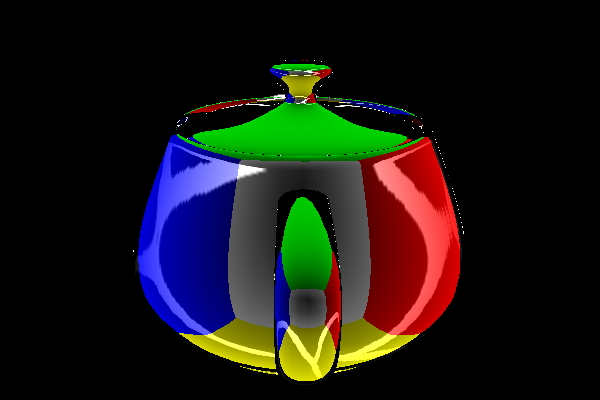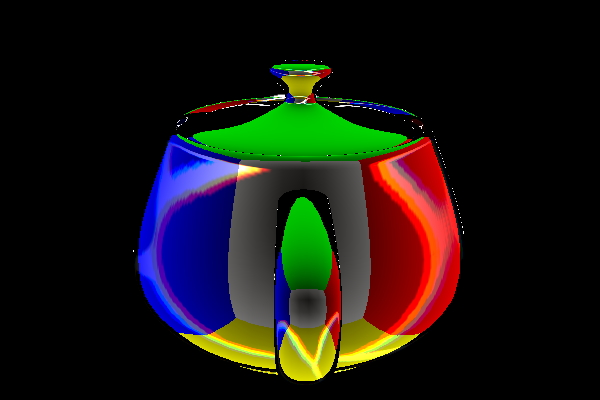Procedural Textures (e.g. the brick example we did before)
• have low memory requirements
• have no fixed resolution
• can be parameterized
but
• may be hard to program
• can be slow depending on the algorithm
• suffer from aliasing (unless you use a more sophisticated/slower algorithm)
May also have combinations of different texturing on the same object

Bump Mapping

modulating the surface normal before applying lighting

adds apparent geometric complexity during fragment processing
lighting will be done in the fragment shader

need normal, light source direction, and viewing direction for each fragment
going to use surface local coordinate space (tangent space)

the point we are working on is defined as (0, 0, 0) and unperturbed normal is (0, 0, 1)

then we have:
- X axis - tangent vector - {1.0, 0.0, 0.0}
- Z axis - normal vector - {0.0, 0.0, 1.0}
- Y axis - binormal vector - {0.0, 1.0, 0.0}  derived from tangent and normal vectors

Which means we now need to convert the light from eye/camera space to tangent space
x = light dot t
y = light dot b
z = light dot n

but before we can do that we need to get t, b, and n.
We have N. We need to pass in a tangent vector as an attribute variable, then we can do:
vec3 n = normalize(gl_NormalMatrix * gl_Normal);
vec3 t = normalize(gl_NormalMatrix * Tangent);
vec3 b = cross(n, t);

A good discussion can be found at: http://www.ozone3d.net/tutorials/bump_mapping.php along with vertex and fragment shader code.

A relevent discussion on normal maps/bump maps at: http://www.ozone3d.net/tutorials/normal_map.php

A normal map is a texture map where each element contains an XYZ normal vector instead of an RGB colour.  A given polygon has a texture map to give the surface colour, and a normal map to give the surface roughness., this way light will appear to reflect off a rough (more interesting and detailed) surface rather than a smooth polygonal surface.

These normal vectors are given in tangent space. Since a texture map can only hold positive values, we need a way to encode negative values, so normals that range from -1 to 1 are represented by texels that range from 0 to 1. A normal map that does nothing would have normals of (0.0, 0.0, 1.0) and to represent that each texel would contain (0.5, 0.5, 1.0) as shown below.  We take the normal data, add 1, and divide by 2. Since the normal maps tend to have strong Z values they will tend to be light bluish.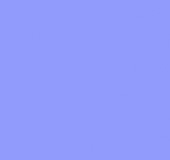When a texel is read from the normal map it needs to be converted back into a real normal (multiply by 2 and subtract 1) and then the vector should be normalized.

Information on tangent space can be found  at:

So lets say we have the following normal map. There are various freeware programs out there that will create normal maps from common image formats.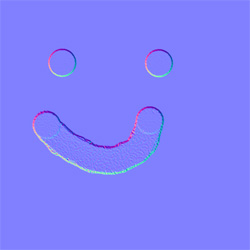// Vertex shader for procedural bumps
// Authors: Randi Rost, John Kessenich
// Copyright (c) 2002-2005 3Dlabs Inc. Ltd.

varying vec3 LightDir;
varying vec3 EyeDir;
varying vec2 TexCoord;

uniform vec3 LightPosition;

attribute vec3 Tangent; // sending in the tangent vector from the main program

void main(void)
{
EyeDir = vec3 (gl_ModelViewMatrix * gl_Vertex);

gl_Position = ftransform();

TexCoord = gl_MultiTexCoord0.st;

// convert normal and tangent (from main program) into eye space
vec3 n = normalize(gl_NormalMatrix * gl_Normal);
vec3 t = normalize(gl_NormalMatrix * Tangent);

// compute b in eye space
vec3 b = cross(n, t);

// convert light direction from eye space to tangent space
vec3 v;
v.x = dot(LightPosition, t);
v.y = dot(LightPosition, b);
v.z = dot(LightPosition, n);
LightDir = normalize(v);

// convert eye direction from eye space to tangent space
v.x = dot(EyeDir, t);
v.y = dot(EyeDir, b);
v.z = dot(EyeDir, n);
EyeDir = normalize(v);
}

// Fragment shader for procedural bumps
// Authors: Randi Rost, John Kessenich
// Copyright (c) 2002-2005 3Dlabs Inc. Ltd.
// tweaked a bunch by Andy

varying vec3 LightDir; // interpolated in tangent space
varying vec3 EyeDir;   // interpolated in tangent space
varying vec2 TexCoord; // interpolated across the normal map

vec3 SurfaceColor = vec3(0.7, 0.6, 0.18); // golden colour for the surface
float SpecularFactor = 0.5;

uniform sampler2D normalName; // sampler for the normal map

void main (void)
{
vec3 litColor;

// use the interpolated TexCoord to read the normal from the normal map
vec3 normDelta = vec3 (texture2D (normalName, TexCoord.st));

// convert the normal from 0.0 - 1.0 to -1.0 - 1.0
normDelta *= 2.0;
normDelta -= 1.0;

// use this new normal to work out the diffuse lighting component
litColor = SurfaceColor * max(dot(normDelta, LightDir), 0.0);

vec3 reflectDir = reflect(LightDir, normDelta);

// use this new normal to work out the specular lighting component
float spec = max(dot(EyeDir, reflectDir), 0.0);
spec = pow(spec, 6.0);
spec *= SpecularFactor;

litColor = min(litColor + spec, vec3 (1.0));

gl_FragColor = vec4 (litColor, 1.0);
}

and this part of the OpenGL application

glUseProgram(program);

GLint texLoc, lightLoc, normalLoc;
texLoc = glGetAttribLocation(program, "Tangent");
glVertexAttrib3f(texLoc, 1.0, 0.0, 0.0);

lightLoc = glGetUniformLocation(program, "LightPosition");
glUniform3f(lightLoc, 1.0, 1.0, 4.0);

normalLoc = glGetUniformLocation(program, "normalName");
glUniform1i(normalLoc, 0);

glNormal3f(0.0f, 0.0f, -1.0f);

glBegin( GL_POLYGON );
glTexCoord2f( 0.0f, 1.0f);
glVertex3f( -2.0f, -2.0f, 0.0f);

glTexCoord2f( 1.0f, 1.0f);
glVertex3f(  2.0f, -2.0f, 0.0f);

glTexCoord2f( 1.0f, 0.0f);
glVertex3f(  2.0f,  2.0f, 0.0f);

glTexCoord2f( 0.0f, 0.0f);
glVertex3f( -2.0f,  2.0f, 0.0f);
glEnd();

which together generates:

So now lets try a procedural bump map from chapter 11 of the Orange Book.

the vertex shader is the same

unlike the previous example, in this case the 'bumps' are not coming from a normal map, but are being computed in the fragment shader based on a bump density and a bump size, so its simular to the way we consturcted the procedural bricks as a texture, except this time we are constructing procedural bumps in the surface.

// Fragment shader for procedural bumps
// Authors: Randi Rost, John Kessenich
// Copyright (c) 2002-2005 3Dlabs Inc. Ltd.
// tweaked a bit by Andy to remove the uniform variables

varying vec3 LightDir;
varying vec3 EyeDir;
varying vec2 TexCoord;

vec3 SurfaceColor = vec3(0.7, 0.6, 0.18);
float BumpDensity = 16.0; // how many bumps in a row or column
float BumpSize    = 0.15; // how big each individual bump is
float SpecularFactor = 0.5;

void main (void)
{
vec3 litColor;
vec2 c = BumpDensity * TexCoord.st; // bump number - c ranges from 0 to 16 in this case
vec2 p = fract(c) - vec2 (0.5); // angle of normal changing over the bump area
// p ranges from -0.5 to 0.5 for each bump, bump centered at 0.0

float d, f;
d = p.x * p.x + p.y * p.y; // d is the 'radius' of this fragment from center of its bump
f = 1.0 / sqrt(d + 1.0);  // f normalizes length of the normal: sqrt(x^2 + y^2 + z^2)

// if we are outside the spherical area of a bump then normal points straight out Z w/ length 1
if (d >= BumpSize)
{
p = vec2(0.0);
f = 1.0;
}

// create a new normal of length 1 for this fragment
vec3 normDelta = vec3 (p.x, p.y, 1.0) * f;

litColor = SurfaceColor * max(dot(normDelta, LightDir), 0.0);
vec3 reflectDir = reflect(LightDir, normDelta);

float spec = max(dot(EyeDir, reflectDir), 0.0);
spec = pow(spec, 6.0);
spec *= SpecularFactor;
litColor = min(litColor + spec, vec3 (1.0));

gl_FragColor = vec4 (litColor, 1.0);
}

and then here is a short bit of code from the OpenGL program.

glUseProgram(program);

GLint texLoc, lightLoc;
texLoc = glGetAttribLocation(program, "Tangent");
glVertexAttrib3f(texLoc, 1.0, 0.0, 0.0);

lightLoc = glGetUniformLocation(program, "LightPosition");
glUniform3f(lightLoc, 1.0, 1.0, 4.0);

glNormal3f(0.0f, 0.0f, -1.0f );

glBegin( GL_POLYGON );
glTexCoord2f( 0.0f, 1.0f );
glVertex3f( -2.0f, -2.0f, 0.0f );

glTexCoord2f( 1.0f, 1.0f );
glVertex3f(  2.0f, -2.0f, 0.0f );

glTexCoord2f( 1.0f, 0.0f );
glVertex3f(  2.0f,  2.0f, 0.0f );

glTexCoord2f( 0.0f, 0.0f );
glVertex3f( -2.0f,  2.0f, 0.0f );
glEnd();

Together this all generates the following:

Here is some sample code for bump mapping: application, vertex shader, fragment shader
and here is the gzipped tar file

Noise
can't be random since we need to repeatedly produce the same pattern each frame

ideally:
• continuous
• repeatable
• well defined output range
• does not show regular patterns
• isotropic (rotationally invariant)
• can be defined for different dimensions
creating a noise texture (chapter 15 of the Orange Book 2nd ed)

GLSL has (will have) a built-in noise function that you can use, as in the code below, once various card makers get around to actually implementing it, until then you need to make your own. The main idea here is to generate noise with courser and finer features that can be added together to create more realistic noise.

Given a starting (course) frequency and amplitude, we create multiple (finer) octaves by doubling the frequency and halving the amplitude in the OpenGL program. We do this once at the beginning of the program execution and store the values in a 3D texture map. The fragment shader can then combine these different levels of noise in different ways to create different materials.

The orange book has some nice figures in chapter 15.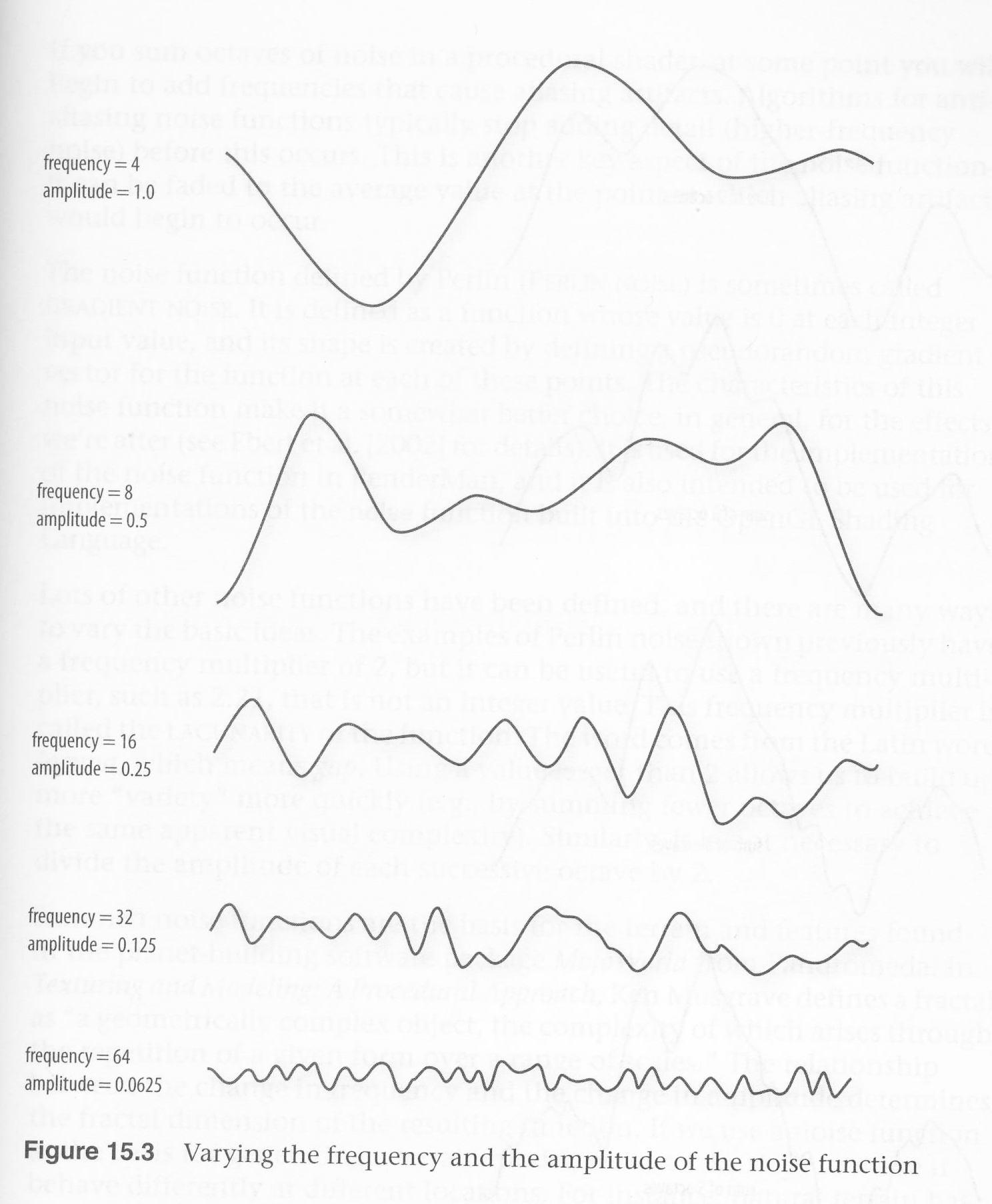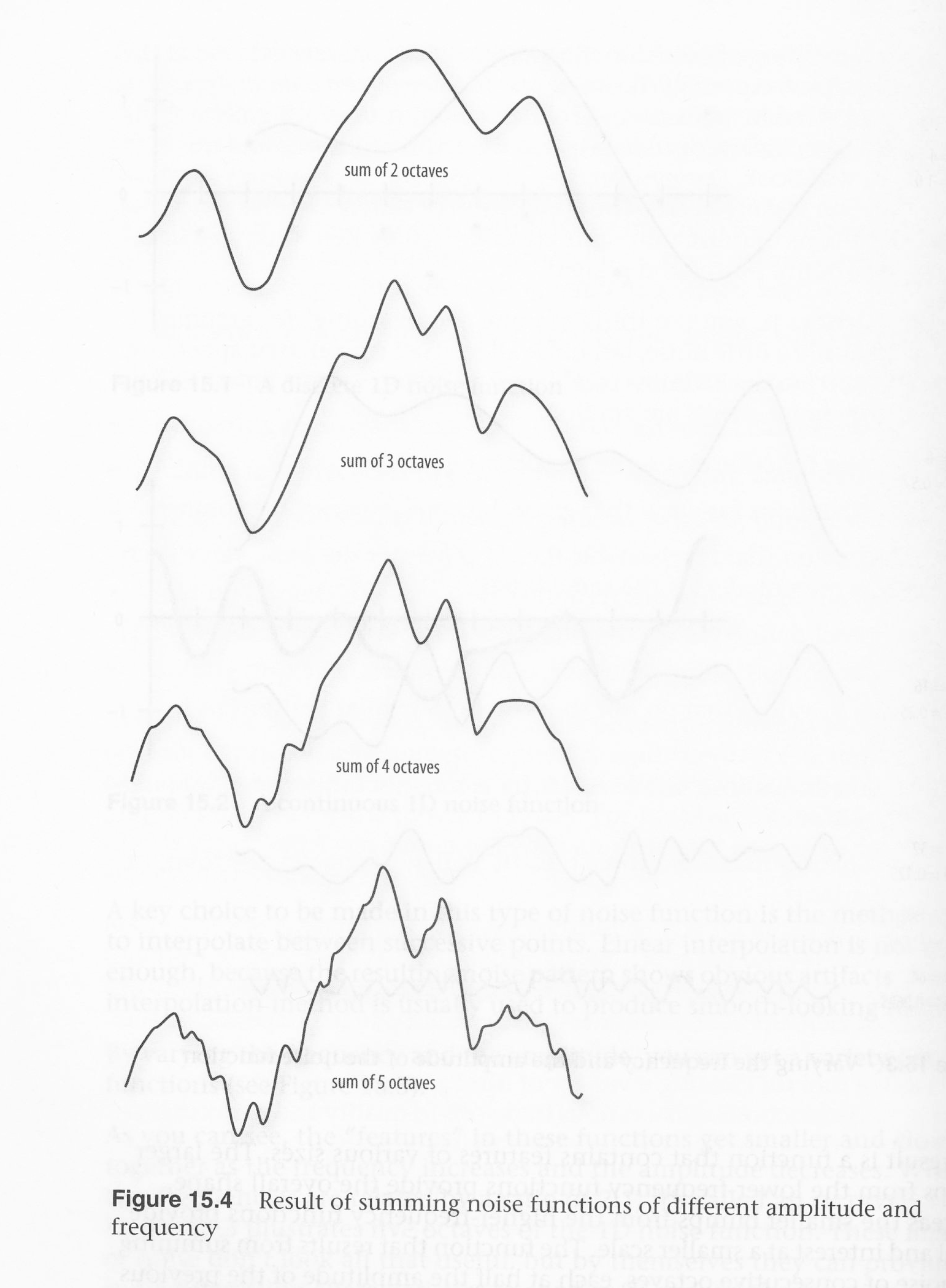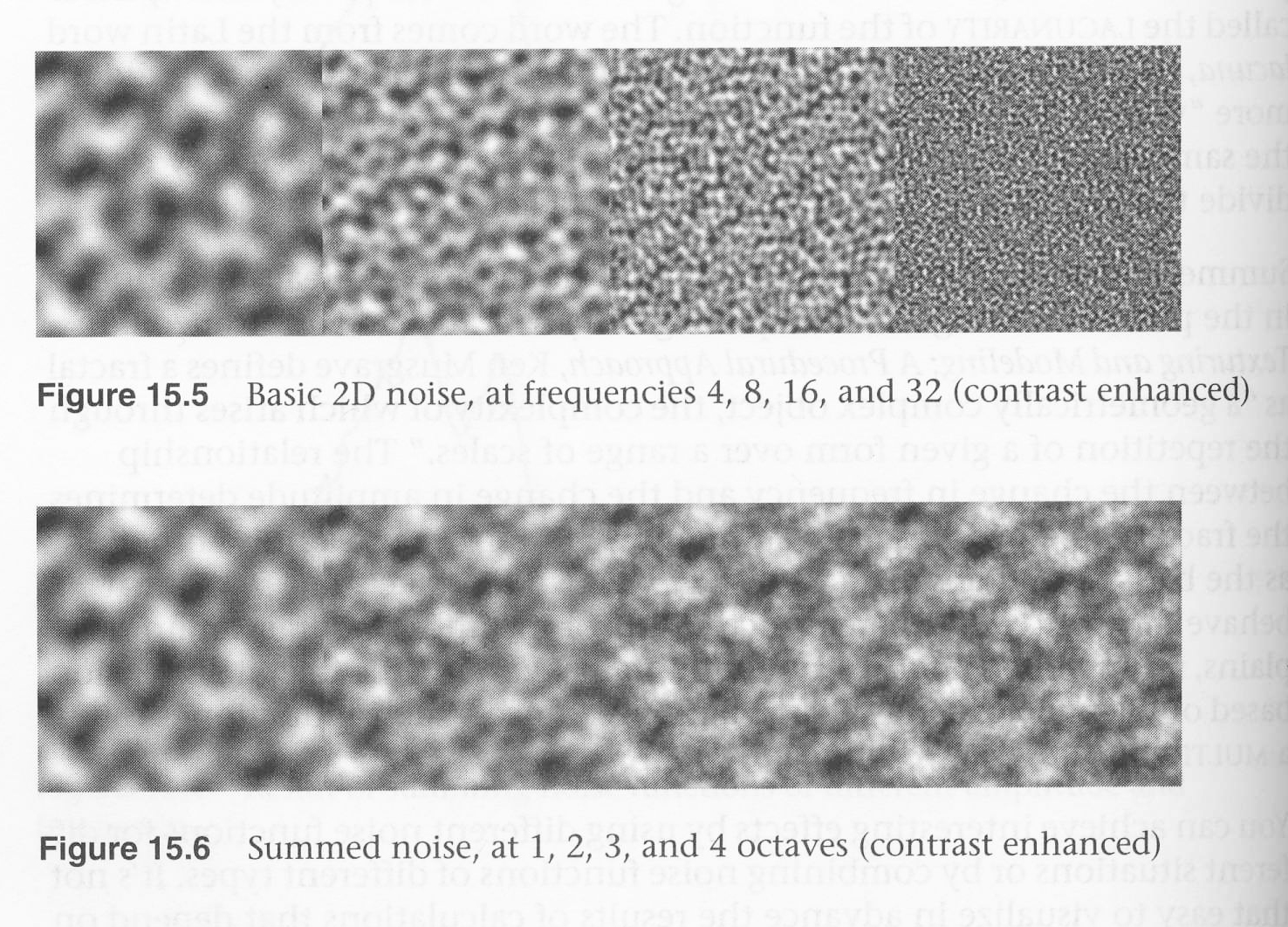int noise3DTexSize = 128;
GLuint noise3DTexName = 0;
GLubyte *noise3DTexPtr;

void make3DNoiseTexture(void)
{
int f, i, j, k, inc;
int startFrequency = 4;
int numOctaves = 4;
double ni;
double inci, incj, inck;
int frequency = startFrequency;
GLubyte *ptr;
double amp = 0.5;

// allocate space for 4 octaves each of size 128 by 128
if ((noise3DTexPtr = (GLubyte *) malloc(noise3DTexSize *
noise3DTexSize *
noise3DTexSize * 4)) == NULL)
{
fprintf(stderr, "ERROR: Could not allocate 3D noise texture\n");
exit(1);
}

// Generate each octave in turn
for (f=0, inc=0; f < numOctaves;
++f, frequency *= 2, ++inc, amp *= 0.5)
{
SetNoiseFrequency(frequency);
ptr = noise3DTexPtr;
ni = ni = ni = 0;

inci = 1.0 / (noise3DTexSize / frequency);
for (i=0; i<noise3DTexSize; ++i, ni += inci)
{
incj = 1.0 / (noise3DTexSize / frequency);
for (j=0; j<noise3DTexSize; ++j, ni += incj)
{
inck = 1.0 / (noise3DTexSize / frequency);
for (k=0; k<noise3DTexSize; ++k, ni += inck, ptr+= 4)
{
*(ptr+inc) = (GLubyte) (((noise3(ni)+1.0) * amp)*128.0);
}
}
}

}
}

We
want a noise function that has features of various sizes and that can be used for multiple types of noise. We are going to store the noise in a 3D texture where each texel contains different octaves in the r, g, b, and a components (each component having twice the frequency and half the amplitude compared to the one before it.)

Perlin has a page on noise here: http://www.noisemachine.com/talk1/
and his original code is available from http://paulbourke.net/texture_colour/perlin/

if you want a newer/better noise algorithm then check out:
http://www.itn.liu.se/~stegu/simplexnoise/simplexnoise.pdf

and, one last link ... there is a nice image here on some of the different effects that are possible:
http://www.noisemachine.com/talk1/19.html

in the vertex shader - clouds:

varying float LightIntensity;
varying vec3  MCposition;

vec3 LightPos = vec3(1.0, 1.0, 1.0);
float Scale = 1.0;

void main(void)

vec3 ECposition = vec3 (gl_ModelViewMatrix * gl_Vertex);
MCposition      = vec3 (gl_Vertex);
vec3 tnorm      = normalize(vec3 (gl_NormalMatrix * gl_Normal));
LightIntensity  = dot(normalize(LightPos - ECposition), tnorm);
gl_Position     = ftransform();
}

in the fragment shader - clouds:

varying float     LightIntensity;
varying vec3      MCposition;

uniform sampler3D Noise;
vec3      SkyColor   = vec3(0.0, 0.0, 0.8);
vec3      CloudColor = vec3(0.8, 0.8, 0.8);

void main(void)
{
vec4  noisevec  = texture3D(Noise, MCposition);
float intensity = (noisevec + noisevec +
noisevec + noisevec + 0.03125) * 1.5;

vec3 color   = mix(SkyColor, CloudColor, intensity) * LightIntensity;

gl_FragColor = vec4(color, 1.0);
}

and then in the main OpenGL program I create the 3D noise texture:

make3DNoiseTexture();

glGenTextures(1, &noise3DTexName);
glBindTexture(GL_TEXTURE_3D, noise3DTexName);

glTexParameterf(GL_TEXTURE_3D, GL_TEXTURE_WRAP_S, GL_REPEAT);
glTexParameterf(GL_TEXTURE_3D, GL_TEXTURE_WRAP_T, GL_REPEAT);
glTexParameterf(GL_TEXTURE_3D, GL_TEXTURE_WRAP_R, GL_REPEAT);
glTexParameterf(GL_TEXTURE_3D, GL_TEXTURE_MAG_FILTER, GL_LINEAR);
glTexParameterf(GL_TEXTURE_3D, GL_TEXTURE_MIN_FILTER, GL_LINEAR);

glTexImage3D(GL_TEXTURE_3D, 0, GL_RGBA,
noise3DTexSize, noise3DTexSize, noise3DTexSize,
0, GL_RGBA, GL_UNSIGNED_BYTE, noise3DTexPtr);

and then when I'm ready to draw:

glBindTexture(GL_TEXTURE_3D, noise3DTexName);

glUseProgram(program);

// we pass the texture in the same as before

GLint texLoc;
texLoc = glGetUniformLocation(program, "Noise");
glUniform1i(texLoc, 0);

glutSolidTeapot(1);

to get

Turbulence / Granite / Wood

use variants of the noise function

in the fragment shader - sun:

varying float     LightIntensity;
varying vec3      MCposition;

uniform sampler3D Noise;
vec3      Color1 = vec3(0.8, 0.7, 0.0);
vec3      Color2 = vec3(0.6, 0.1, 0.0);

void main(void)
{
vec4 noisevec = texture3D(Noise, MCposition);
float intensity = abs(noisevec - 0.25) +
abs(noisevec - 0.125) +
abs(noisevec - 0.0625) +
abs(noisevec - 0.03125);

intensity    = clamp(intensity * 6.0, 0.0, 1.0);
vec3 color   = mix(Color1, Color2, intensity) * LightIntensity;
gl_FragColor = vec4(color, 1.0);
}

to get:

in the fragment shader - marble:

varying float     LightIntensity;
varying vec3      MCposition;

uniform sampler3D Noise;
vec3      MarbleColor = vec3(1.0, 1.0, 1.0);
vec3      VeinColor = vec3(0.1, 0.8, 0.2);

void main(void)
{
vec4  noisevec  = texture3D(Noise, MCposition);
float intensity = abs(noisevec - 0.25) +
abs(noisevec - 0.125) +
abs(noisevec - 0.0625) +
abs(noisevec - 0.03125);

float sineval = sin(MCposition.y * 6.0 + intensity * 12.0) * 0.5 + 0.5;
vec3  color   = mix(VeinColor, MarbleColor, sineval) * LightIntensity;
gl_FragColor  = vec4(color, 1.0);
}

to get: